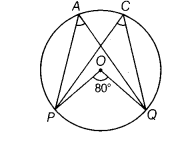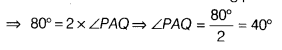# In the given figure, if ∠POQ = 80°, then find ∠PAQ and∠PCQ

In the given figure, if ∠POQ = 80°, then find ∠PAQ and ∠PCQ.Given, ∠POQ = 80°
From the given figure, we have ∠POQ =2 x ∠PAQ

[∴ angle subtended by an arc at the centre is double the angle subtended by it at any point on the remaining part of the circle]Now ∠PCQ =∠PAQ
[ ∴ angle in the same segment are equal]

∠PCQ = 40°